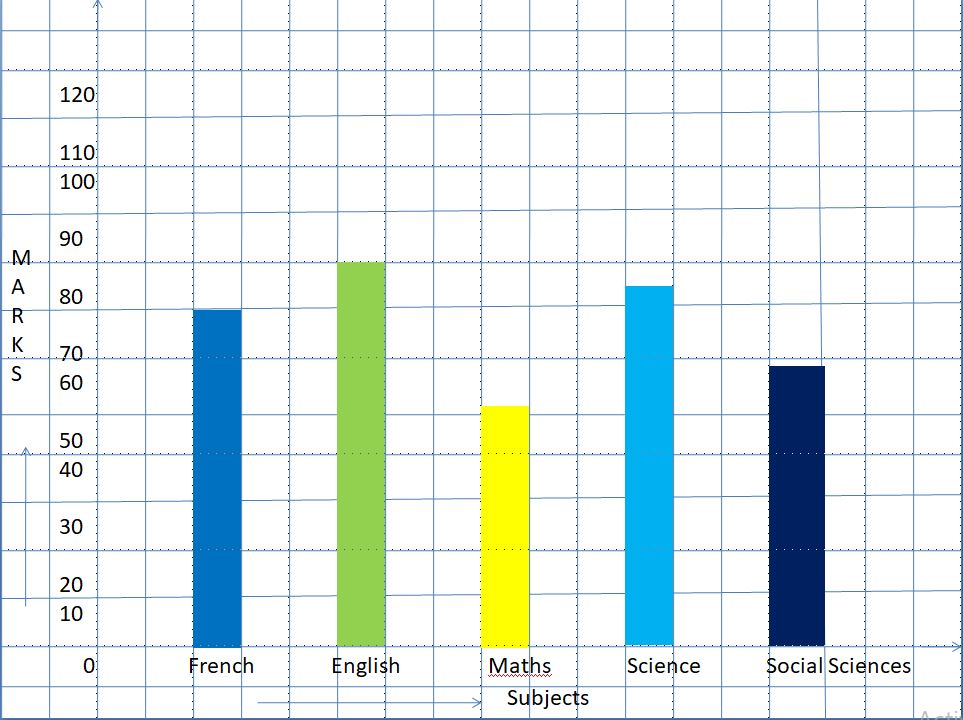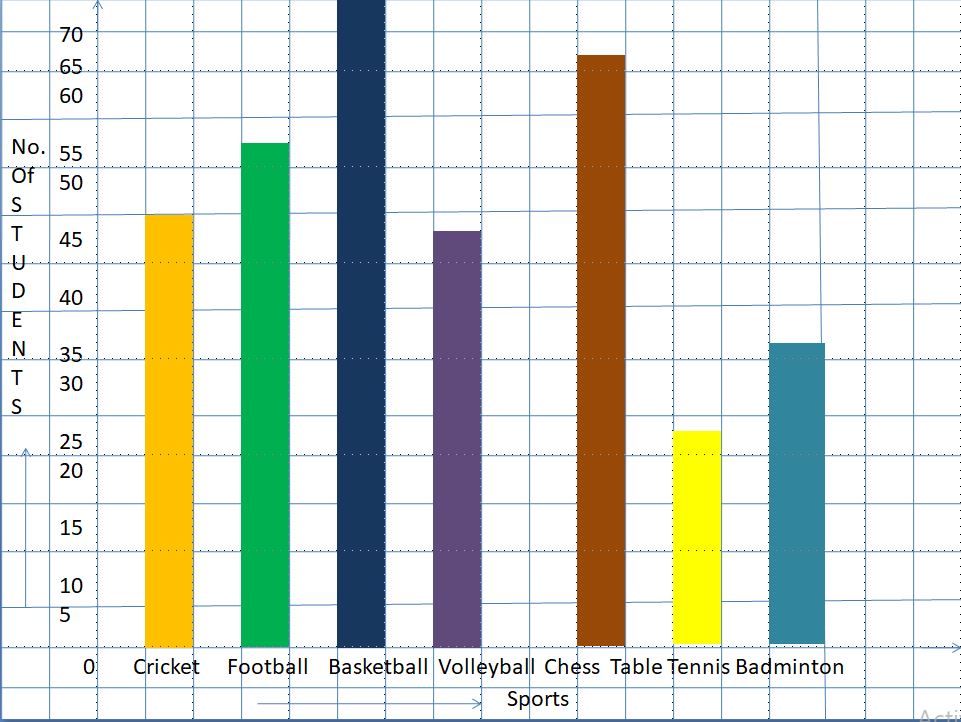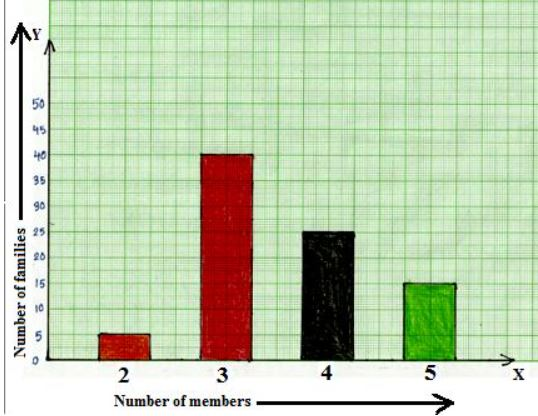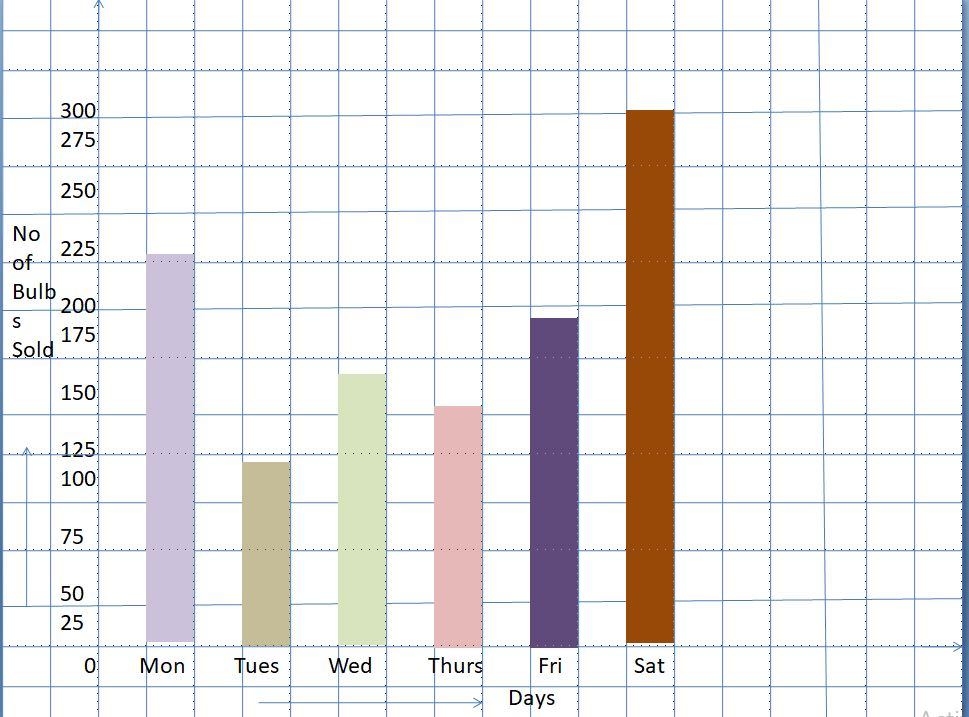# Worksheet on Bar Graphs or Column Graphs | Bar Graph Examples with Question and Answers

Worksheet on Bar Graphs or Column Graphs has the questions on representing the data as a bar graph. To represent the data as rectangular bars, we need to take data along the x-axis, y-axis. And select a suitable scale, draw the rectangular bars. The pictorial representation of grouped data as horizontal or vertical bars is called the bar graph. In the below sections of this page, we are giving the questions and answers related to the bar graphs or column graphs. Solve them and learn different types of questions in Bar Graph.

1. The marks obtained by Aravind in his annual examination are shown below:

Subject French English Maths Science Social Sciences
Marks 70 80 50 75 60

Draw a bar graph chart to represent the above data.

Solution:

Given that,

The marks obtained by Aravind in the annual examination.

On graph paper, draw horizontal and vertical lines.

Take subjects along the x-axis at equal gaps, marks along the y-axis.

Choose 1 small division = 10 units

Then the heights of the bars are:

1 small division = 10 dollars

Then, the heights of the bars are:

French marks = (1/10 * 70) = 7 small divisions

Marks in English = (1/10 * 80) = 8 small divisions

Marks in Maths = (1/10 * 50) = 5 small divisions

Marks in Science = (1/10 * 75) = 7.5 small divisions

Marks in Social Sciences = (1/10 * 60) = 6 small divisionsBar graph showing Aravind marks in Annual Examination

At the points marked in Step 2, draw bars of equal width and of heights calculated.

2. The population in lakhs of seven Indian states as estimated in 2010 is given below:

Population (in Lakhs) 520 400 250 800 750 690

Represent the above data by a bar graph.

Solution:

Given that,

The population of seven Indian states estimated in 2010.

Draw horizontal, vertical lines on a graph paper.

Take states along the x-axis at equal gaps, the population in lakhs along the y-axis.

Choose 1 small division = 100 lakhs

Then, the heights of the bars are:

Population in Andhra Pradesh = (1/100 * 520) = 5.2 small divisions

Population in Assam = (1/100 * 400) = 4 small divisions

Population in Bihar = (1/100 * 250) = 2.5 small divisions

Population in Telangana = (1/100 * 800) = 8 small divisions

Population in Tamil Nadu = (1/100 * 750) = 7.5 small divisions

Population in Rajasthan = (1/100 * 690) = 6.8 small divisionsBar graph showing the population in seven Indian states.

3. A school conducted a survey to know the favorite sports of the students. The table below shows the results of this survey.

Name of the Sport Cricket Football Basketball Volleyball Chess Table Tennis Badminton
Total Number of Students 45 53 70 44 66 22 33

From this data,

(a) Draw a graph representing the sports and the total number of students.

(b) Which sport is the most preferred one?

(c) List the sports in ascending order.

(d) Which two sports are almost equally preferred?

(e) Calculate the range of the graph.

Solution:

The favorite sports of students according to the survey are given.

(a) On a graph paper, draw a horizontal line OX and a vertical line OY, representing the x-axis and the y-axis respectively.

Take Sport name along the x-axis, the total number of students along the y-axis.

On a scale, choose 1 small division = 5 units

Number of students whose favorite game cricket = (1/5 * 45) = 9 small divisions

Number of students whose favorite game football = (1/5 * 53) = 10.6 small divisions

Number of students whose favorite game basketball = (1/5 * 70) = 14 small divisions

Number of students whose favorite game volleyball = (1/5 * 44) = 8.8 small divisions

Number of students whose favorite game chess = (1/5 * 66) = 13.2 small divisions

Number of students whose favorite game tabletennis = (1/5 * 22) = 4.4 small divisions

Number of students whose favorite game badminton = (1/5 * 33) = 6.6 small divisionsBar graph showing students favorite game

From the graph,

(b) The most preferred sport is basketball.

(d) Cricket, Volleyball are two sports that are almost equally preferred.

(e) The range of the graph is 70 – 22 = 48

4. The number of cars produced in a factory during five consecutive weeks is given below:

Week First Second Third Fourth Fifth
Number of Cars Produced 500 800 1060 1400 1700

Draw a bar graph or column graph representing the above information.

Solution:

Given that,

The number of cars produced in a factory for five consecutive weeks.

Draw two perpendicular lines, x-axis, y-axis on a graph paper.

Take weeks along the x-axis at equal gaps.

Take the number of cars produced per week along the y-axis.

Scale on the y-axis is 1 small division = 200 cars.

According to the scale, draw rectangular bars for each week.

Fill color in it to show the variation.Column graph or bar graph representing the number of cars produced by a compy for five consecutive weeks.

5. A survey of 145 people asked them “Which is the nicest fruit?”

Fruit Apple Orange Banana Kiwifruit Blueberry Grapes
People 35 30 10 25 40 5

Draw a bar graph for the data.

Solution:

Draw two perpendicular lines on a graph paper.

Take fruit on the x-axis, people number on the y -axis.

Choose 1 small division = 5 units

Then, the heights of the bars are:

Number of people who like apple = (1/5 * 35) = 7 small divisions

Number of people who like orange = (1/5 * 30) = 6 small divisions

Number of people who like banana = (1/5 * 10) = 2 small divisions

Number of people who like kiwifruit = (1/5 * 25) = 5 small divisions

Number of people who like blueberry = (1/5 * 40) = 8 small divisions

Number of people who like grapes = (1/5 * 5) = 1 small divisionsThe nicest fruit according to the survey is blueberry.

6. In a survey of 85 families of a colony, the number of members in each family was recorded, and the data has been represented by the following bar graph.(i) What information does the bar graph give?

(ii) How many families have 3 members?

(iii) How many people live alone?

(iv) Which type of family is the most common? How many members are there in each family of this kind?

Solution:

From the given graph we can observe that,

(i) The graph tells about the number of families containing 2, 3, 4, 5 members each.

(ii) 40 families have 3 members.

(iii) No one lives alone.

(iv) Family having 3 members is the most common type.

7. The following graph shows the life expectancy (average age to which people live) in various countries in a particular year.(i) What information does the bar graph give?

(ii) Which country has the highest, lowest life expectancy?

(iii) What is the life expectancy in India?

(iv) What is the range of the graph?

Solution:

The given graph represents the life expectancy of various countries.

From the given graph we can observe that,

(i) Graph tells about the life expectancy of five different countries in a particular year.

(ii) Japan has the highest life expectancy which is 70 years. Cambodia has the lowest life expectancy which is 40 years.

(iii) India life expectancy is 63 years.

(iv) Range is 70 – 40 = 30.

8. The following table shows the market position of different types of lotions

Brands A B C D E others
Percentage of Buyer 50 20 40 30 60 10

Represent the given data in a bar graph or column graph.

Solution:

Given that,

The market position of different types of lotions.

On a graph paper, draw a horizontal line OX and a vertical line OY, representing the x-axis and the y-axis respectively.

Take brands along the x-axis at equal gaps, percentage of buyers along the y-axis.

Scale, choose 1 small division = 5 units

Then, the heights of the bars are:

Brand A position in market = (1/5 * 50) = 10

Brand B position in market = (1/5 * 20) = 4

Brand C position in market = (1/5 * 40) = 8

Brand D position in market = (1/5 * 30) = 6

Brand E position in market = (1/5 * 60) = 12

Other brand position in market = (1/5 * 10) = 2Bar graph showing the brands position in the market.

9. Given below is a graph showing the number of electric bulbs sold in a shop for a week.Read the bar graph or column graph carefully and answer the questions given below:

(i) On which day of the week was the sale minimum?

(ii) On which day of the week was the sale maximum?

(iii) What was the total sale during the week?

(iv) What is the ratio between the minimum sale and the maximum sale?

Solution:

given graph shows the number of electric bulbs sold in a shop for a week.

By observing the graph,

(i) On Tuesday, the sale was minimum.

(ii) On Saturday, the sale was maximum.

(iii) The sale during the week is 1040 bulbs.

(iv) The minimum sale during the week = 100 bulbs.

The maximum sale during the week = 275 bulbs.

Therefore, minimum sale : maximum sale = 100 : 275 = 20 : 55.

10. Various modes of transport used by 1830 students of a school are given below:

School Bus Private Bus Bicycle Rickshaw By Foot
640 220 500 120 350

Draw a bar graph to represent the above data.

Solution:

On a graph paper, take the mode of transport on the x-axis, the number of students on y-axis.

Take 1 small division = 50 students

Then, the heights of the bars are:

Number of students used school bus = (1/ 50 * 640) = 12.8

Number of students used bicycle = (1/ 50 * 500) = 10

Number of students used private bus = (1/ 50 * 220) = 4.4

Number of students used rickshaw = (1/ 50 * 120) = 2.4

Number of students reach school by foot = (1/ 50 * 350) = 711. A teacher of elementary school recorded the favorite subjects of her students in a bar graph. Use the graph to answer the following questions.(i) What unit of the scale is used to display the popularity of subjects?

(ii) Which subject is the second most popular?

(iii) Which subject is less popular?

(iv) Which subject is favorite for 20 students?

(v) For how many students, maths is the favorite subject?

Solution:

The given bar graph represents the favorite subjects for the students of a class.

By observing the graph, we can say that

(i) The popularity of the subjects is taken along the x-axis on the bar graph.

(ii) The second most popular subject is social.

(iii) The less popular subject is language.

(iv) Language is a favorite subject for 20 students in the class.

(v) For 40 students maths is the favorite subject.